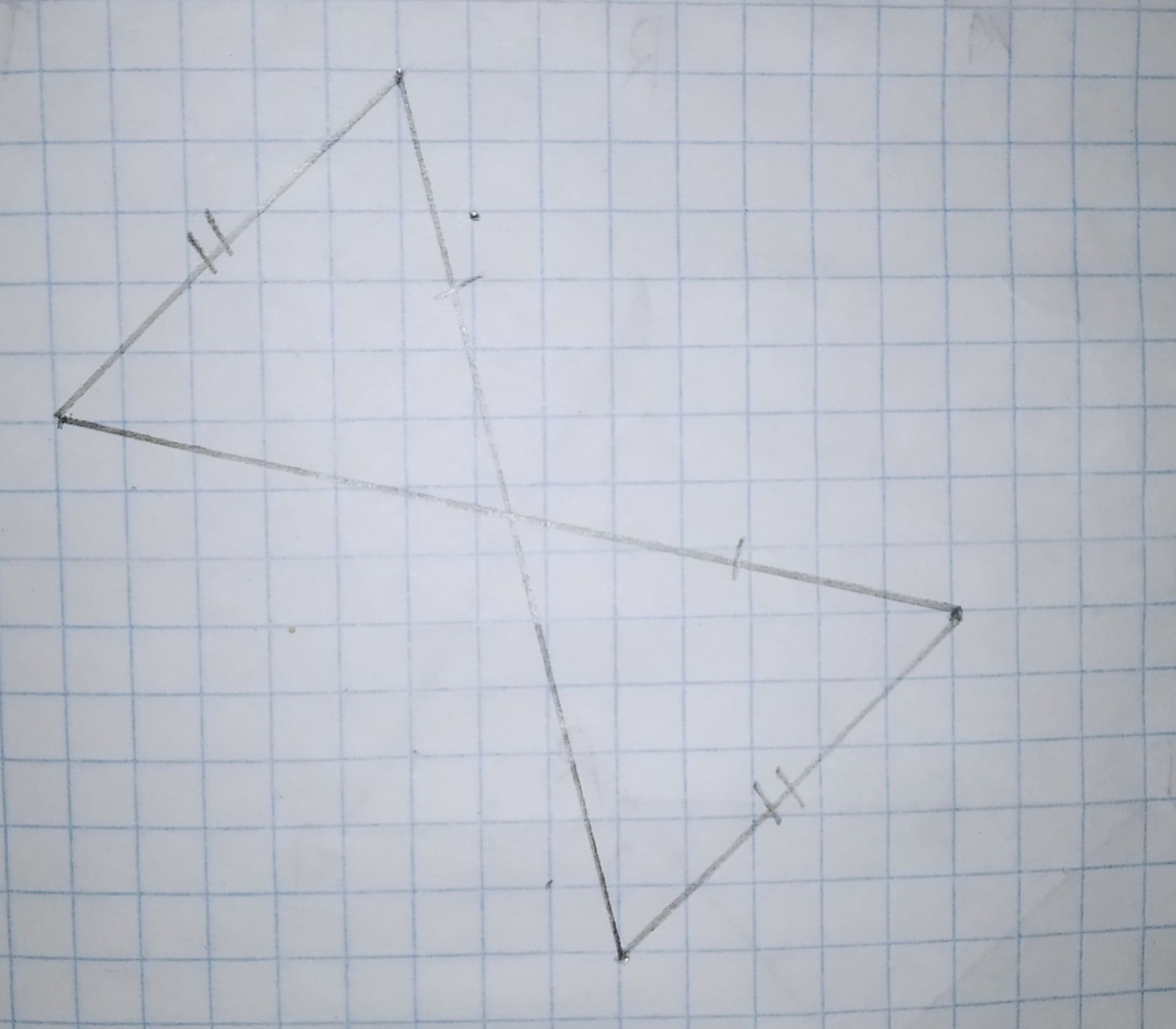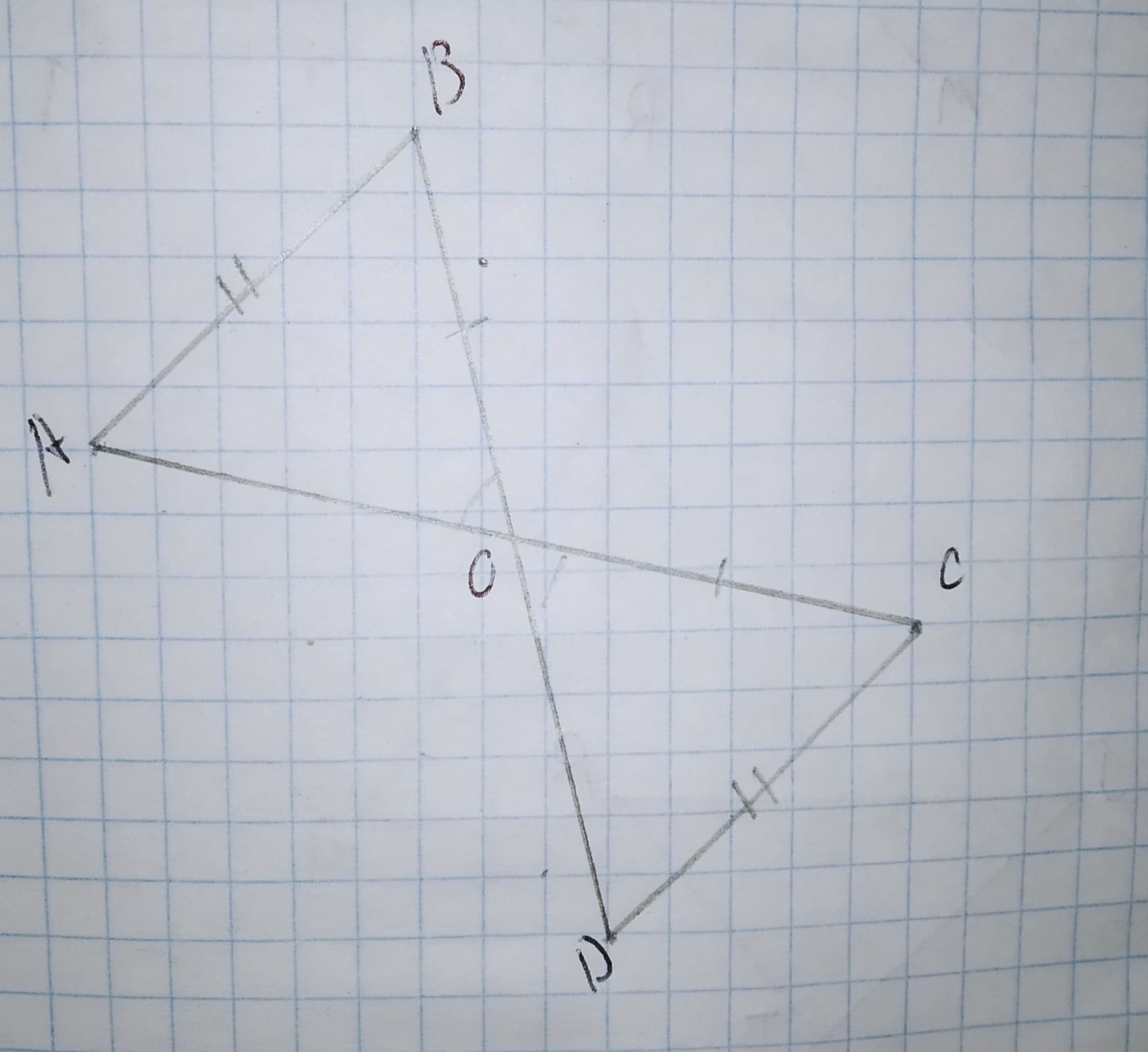Question# decide whether enough information is given to prove that the triangles are congruent using the SAS Congruence Theorem.12210203651.jpg

Congruence
ANSWEREDdecide whether enough information is given to prove that the triangles are congruent using the SAS Congruence Theorem.2021-02-14

Proof of SAS
For SAS theorem , two sides and one angle should be equal in two triangle
then two triangle called congruence,
in this triangle two sides are given .
Step 2For SAS Theorem, Two sides and one angle should be equal in two triangles.
AB = DE (Given in figure)
BC = CE (Given in figure)
$$\displaystyle\angle{A}{C}{B}=\angle{E}{C}{D}$$ [In a pair of intersecting lines the vertically opposite angles are equal]
So $$\displaystyle\triangle{A}{C}{B}\stackrel{\sim}{=}\triangle{E}{C}{D}$$